Electron. J. Differential Equations, Vol. 2016 (2016), No. 266, pp. 1-16.

### Convergence of exterior solutions to radial Cauchy solutions for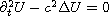Helge Kristian Jenssen, Charis Tsikkou

Abstract:
Consider the Cauchy problem for the 3-D linear wave equationwith radial initial data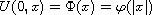,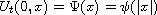. A standard result states that U belongs to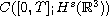whenever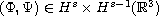. In this article we are interested in the question of how U can be realized as a limit of solutions to initial-boundary value problems on the exterior of vanishing balls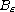about the origin. We note that, as the solutions we compare are defined on different domains, the answer is not an immediate consequence of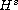well-posedness for the wave equation. We show how explicit solution formulae yield convergence and optimal regularity for the Cauchy solution via exterior solutions, when the latter are extended continuously as constants onat each time. We establish that for s=2 the solution U can be realized as an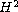-limit (uniformly in time) of exterior solutions on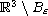satisfying vanishing Neumann conditions along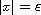, as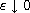. Similarly for s=1: U is then an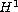-limit of exterior solutions satisfying vanishing Dirichlet conditions along.

Submitted July 22, 2016. Published September 30, 2016.
Math Subject Classifications: 35L05, 35L15, 35L20.
Key Words: Linear wave equation; Cauchy problem; radial solutions; exterior solutions; Neumann and Dirichlet conditions.

Show me the PDF file (283 KB), TEX file for this article.Helge Kristian Jenssen Department of Mathematics Penn State University, University Park State College, PA 16802, USA email: jenssen@math.psu.edu Charis Tsikkou Department of Mathematics West Virginia University Morgantown, WV 26506, USA email: tsikkou@math.wvu.edu